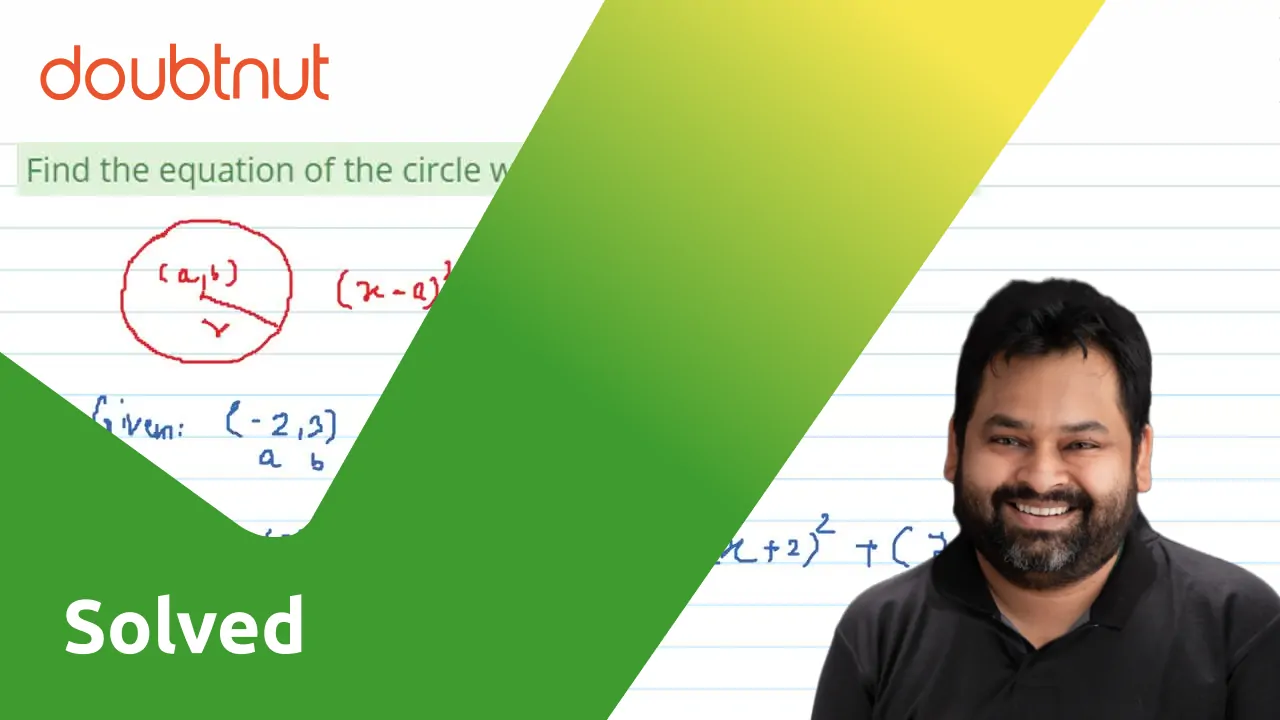Doubtnut App - Get 50 Lakh+ video solutions better than GoogleInstall Now

# Find the equation of the circle with: centre (-2,3) and radius 4.

Text Solution
Verified by Experts

## (##XI11_E0102_##) Here it is given that center (h,k)=(−2,3) and radius r=4 ∴ the equation of the circle is (x+2)2+(y−3)2=42 =>x2+4x+4+y2−6y+9=16 =>x2+y2+4x−6y−3=0